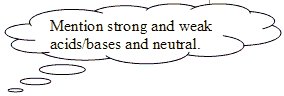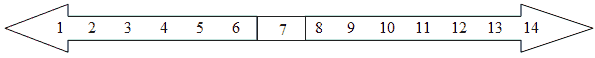# The Material World: Chemistry

 Page 7/7 Date 23.04.2018 Size 478.7 Kb. #45992

(A, M)

1. Discuss what an ion is and why one forms. Mention the names for negative and positive ions.

(M)

1. Explain why Nobel gases do not react in terms of valence electrons.

(E)

1. Acids and bases are special compounds with distinct characteristics. State whether the following compounds are acids or bases. (A, M)
HCl _________ NaOH _________ CaCO3 _________
KOH _________ Na2CO3 __________ H2SO4 _________
HNO3 _________ Mg(OH)2 __________ Al2S3_________

Circle the correct answer for the following questions.

1. Which term refers to the amount of water present in a mixture of acid and water?
A. strength B. concentration C. power D. quantity

2. Weak, dilute acids are contained in some foods. What do acids taste like?
A. bitter B. salty C. sweet D. sour

3. Which of the following is a base?
A. baking soda B. lemon juice C. vinegar D. water

4. Which of the following is an acid?
A. cooking oil B. lemon juice C. baking soda D. Janola

5. Which of these gases relights a glowing ember?
A. hydrogen B. carbon dioxide C. oxygen D. nitrogen

6. Which of thee gases gives a "POP" reaction?
A. hydrogen B. carbon dioxide C. oxygen D. nitrogen

7. Which of the following would neutralise sulphuric acid?
A. vinegar B. sodium hydroxide C. nitric acid D. water

8. Acids change the colour of litmus paper from:
A. red to blue B. blue to red
C. yellow to blue D. blue to yellow

9. Strong bases change to _____________ when universal indicator is added.
A. green B. purple C. red D. orange

10. Weak acids are _____________ with universal indicator.
A. orange B. red C. green D. blue

(A, M, E)1. Fill in the pattern of colours used to predict pH with universal indicator:(A, M)

1. Complete the table with the formula and name for each compound formed when the ions combine.
 Ions Compound formed Name of compound Na2+ S2- NaS Sodium sulfide H+ NO3- Al3+ O2- Ca2+ OH- Mg2+ SO42- Li+ CO32-

(A, M)

1. State which ions are attracted to form the following compounds:
 Ions Compound K+ Cl- Potassium chloride NaOH Magnesium sulphate Li2 O Calcium nitrate Al2 (CO3 )3

(A, M)

1. Match the following words to their definitions.
 Acid A A permanent change where a new substance is made. Base B A bond where eletrons are shared between nonmetals. Chemical change C A chemical that releases H + ions and tastes sour. Covalent bond D A compound changes state, but can change back. Indicator E A compound added to another, which then reacts. Ionic bond F A chemical that nuetralises an acid. Product G A chemical that can identify acids and bases.. Physical change H Reactant + Reactant Product Reactant I A bond made by attraction of metal to nonmetal. Reaction J A substance made as the result of a reaction.

(A, M)

1. A neutralisation reaction can follow either of these general reaction equations:
Base + Acid Salt + Water
Carbonate + Acid Salt + Carbon dioxide (g) + Water

Complete the following word equations. Keep to the general examples given.

1. Sodium hydroxide + Hydrochloric acid Sodium chloride + ____________

2. Potassium ______________ + Nitric acid Potassium nitrate + Water

3. Calcium carbonate + Hydrochloric acid Calcium chloride + __________ __________(g) + Water

4. ___________________ + Sulfuric acid Magnesium ___________ + Carbon dioxide (g) + Water

(A, M)

1. Complete the following word equations using the general equation for a reaction between a metal and an acid:

Metal + Acid Salt + Hydrogen(g)

1. Iron + Hydrochloric acid ____________(II) ____________ + Hydrogen (g)

2. Magnesium + ______________ acid Magnesium nitrate + ______________

3. ________________ + Ethanoic acid Calcium ethanoate + Hydrogen (g)

4. _______________ + Sulfuric acid Sodium _______________ + Hydrogen (g)

(M, E)

RESOURCES

Electronic

• Science Pages: Science Revision and Resources, Rates of Reaction & Collision Theory
This site has animations to demonstrate several of the factors that affect the rate of a reaction.

• Doc Brown's Chemistry Clinic
The GCSE website provides notes about rates of reactions, as well as worksheets and quizzes to support learning.